### IMO Shortlist 2011 problem G6

Kvaliteta:
Avg: 0,0
Težina:
Avg: 8,0
Let$ABC$ be a triangle with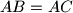$AB=AC$ and let$D$ be the midpoint of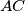$AC$. The angle bisector of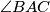$\angle BAC$ intersects the circle through$D,B$ and$C$ at the point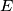$E$ inside the triangle$ABC$. The line$BD$ intersects the circle through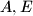$A,E$ and$B$ in two points$B$ and$F$. The lines$AF$ and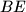$BE$ meet at a point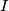$I$, and the lines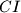$CI$ and$BD$ meet at a point$K$. Show that$I$ is the incentre of triangle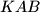$KAB$.

Proposed by Jan Vonk, Belgium and Hojoo Lee, South Korea
Izvor: Međunarodna matematička olimpijada, shortlist 2011